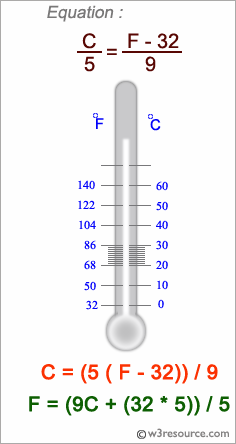﻿ NumPy: Convert the values of Centigrade degrees into Fahrenheit degrees - w3resource# NumPy: Convert the values of Centigrade degrees into Fahrenheit degrees

## NumPy: Array Object Exercise-14 with Solution

Write a NumPy program to convert the values of Centigrade degrees into Fahrenheit degrees. Centigrade values are stored into a NumPy array.
Sample Array [0, 12, 45.21 ,34, 99.91]Sample Solution:-

Python Code:

``````import numpy as np
fvalues = [0, 12, 45.21, 34, 99.91]
F = np.array(fvalues)
print("Values in Fahrenheit degrees:")
print(F)
print(5*F/9 - 5*32/9)
```
```

Sample Output:

```Values in Fahrenheit degrees:
[  0.    12.    45.21  34.    99.91]
[-17.77777778 -11.11111111   7.33888889   1.11111111  37.72777778]
```

Python Code Editor:

Have another way to solve this solution? Contribute your code (and comments) through Disqus.

What is the difficulty level of this exercise?

Test your Python skills with w3resource's quiz

﻿

## Python: Tips of the Day

Set comprehension:

```>>> m = {x ** 2 for x in range(5)}
>>> m
{0, 1, 4, 9, 16}
```